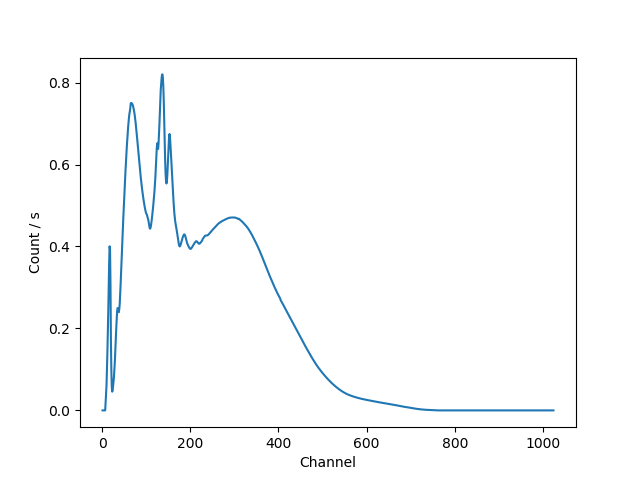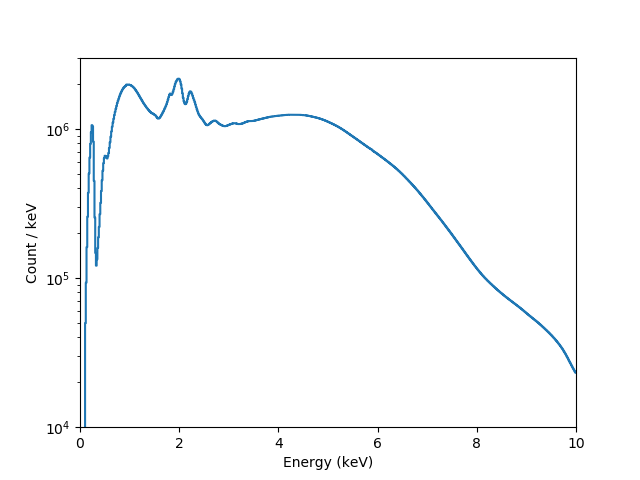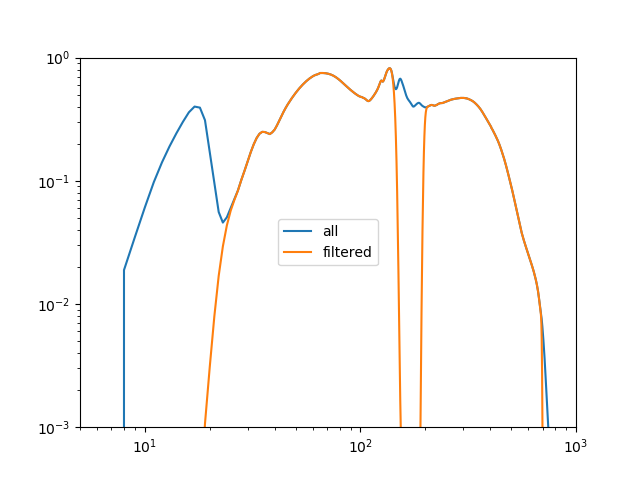# Examples¶

The following examples show the different ways that a model can be evaluted, for a range of situations. The direct method is often sufficient, but for more complex cases it can be useful to ask a data object to evaluate the model, particularly if you want to include instrumental responses, such as a RMF and ARF.

## Evaluating a one-dimensional model directly¶

In the following example a one-dimensional gaussian is evaluated on a grid of 5 points by using the model object directly. The first approch just calls the model with the evaluation grid (here the array x), which uses the parameter values as defined in the model itself:

>>> from sherpa.models.basic import Gauss1D
>>> gmdl = Gauss1D()
>>> gmdl.fwhm = 100
>>> gmdl.pos = 5050
>>> gmdl.ampl = 50
>>> x = [4800, 4900, 5000, 5100, 5200]
>>> y1 = gmdl(x)


The second uses the calc() method, where the parameter values must be specified in the call along with the grid on which to evaluate the model. The order matches that of the parameters in the model, which can be found from the pars attribute of the model:

>>> [p.name for p in gmdl.pars]
['fwhm', 'pos', 'ampl']
>>> y2 = gmdl.calc([100, 5050, 100], x)
>>> y2 / y1
array([ 2.,  2.,  2.,  2.,  2.])


Since in this case the amplitude (the last parameter value) is twice that used to create y1 the ratio is 2 for each bin.

## Evaluating a 2D model to match a Data2D object¶

In the following example the model is evaluated on a grid specified by a dataset, in this case a set of two-dimensional points stored in a Data2D object. First the data is set up (there are only four points in this example to make things easy to follow).

>>> from sherpa.data import Data2D
>>> x0 = [1.0, 1.9, 2.4, 1.2]
>>> x1 = [-5.0, -7.0, 2.3, 1.2]
>>> y = [12.1, 3.4, 4.8, 5.2]
>>> twod = Data2D('data', x0, x1, y)


For demonstration purposes, the Box2D model is used, which represents a rectangle (any points within the xlow to xhi and ylow to yhi limits are set to the ampl value, those outside are zero).

>>> from sherpa.models.basic import Box2D
>>> mdl = Box2D('mdl')
>>> mdl.xlow = 1.5
>>> mdl.xhi = 2.5
>>> mdl.ylow = -9.0
>>> mdl.yhi = 5.0
>>> mdl.ampl = 10.0


The coverage have been set so that some of the points are within the “box”, and so are set to the amplitude value when the model is evaluated.

>>> twod.eval_model(mdl)
array([  0.,  10.,   10.,   0.])


The eval_model() method evaluates the model on the grid defined by the data set, so it is the same as calling the model directly with these values:

>>> twod.eval_model(mdl) == mdl(x0, x1)
array([ True,  True,  True,  True], dtype=bool)


The eval_model_to_fit() method will apply any filter associated with the data before evaluating the model. At this time there is no filter so it returns the same as above.

>>> twod.eval_model_to_fit(mdl)
array([  0.,  10.,   10.,   0.])


Adding a simple spatial filter - that excludes one of the points within the box - with ignore() now results in a difference in the outputs of eval_model() and eval_model_to_fit(), as shown below. The call to get_indep() is used to show the grid used by eval_model_to_fit().

>>> twod.ignore(x0lo=2, x0hi=3, x1l0=0, x1hi=10)
>>> twod.eval_model(mdl)
array([  0.,  10.,   10.,   0.])
>>> twod.get_indep(filter=True)
(array([ 1. ,  1.9,  1.2]), array([-5. , -7. ,  1.2]))
>>> twod.eval_model_to_fit(mdl)
array([  0.,  10.,   0.])


## Evaluating a model using a DataPHA object¶

This example is similar to the two-dimensional case above, in that it again shows the differences between the eval_model() and eval_model_to_fit() methods. The added complication in this case is that the response information provided with a PHA file is used to convert between the “native” axis of the PHA file (channels) and that of the model (energy or wavelength). This conversion is handled automatically by the two methods (the following example shows how this can be done manually).

>>> from sherpa.astro.io import read_pha
statistical errors were found in file '3c273.pi'
but not used; to use them, re-read with use_errors=True
statistical errors were found in file '3c273_bg.pi'
but not used; to use them, re-read with use_errors=True
>>> pha
<DataPHA data set instance '3c273.pi'>
>>> pha.get_arf()
<DataARF data set instance '3c273.arf'>
>>> pha.get_rmf()
<DataRMF data set instance '3c273.rmf'>


The returned object - here pha - is an instance of the sherpa.astro.data.DataPHA class - which has a number of attributes and methods specialized to handling PHA data.

This particular file has grouping information in it, that it it contains GROUPING and QUALITY columns, so Sherpa applies them: that is, the number of bins over which the data is analysed is smaller than the number of channels in the file because each bin can consist of multiple channels. For this file, there are 46 bins after grouping (the filter argument to the get_dep() call applies both filtering and grouping steps, but so far no filter has been applied):

>>> pha.channel.size
1024
>>> pha.get_dep().size
1024
>>> pha.grouped
True
>>> pha.get_dep(filter=True).size
46


A filter - in this case to restrict to only bins that cover the energy range 0.5 to 7.0 keV - is applied with the notice() call, which removes four bins for this particular data set:

>>> pha.set_analysis('energy')
>>> pha.notice(0.5, 7.0)
>>> pha.get_dep(filter=True).size
42


A power-law model (PowLaw1D) is created and evaluated by the data object:

>>> from sherpa.models.basic import PowLaw1D
>>> mdl = PowLaw1D()
>>> y1 = pha.eval_model(mdl)
>>> y2 = pha.eval_model_to_fit(mdl)
>>> y1.size
1024
>>> y2.size
42


The eval_model() call evaluates the model over the full dataset and does not apply any grouping, so it returns a vector with 1024 elements. In contrast, eval_model_to_fit() applies both filtering and grouping, and returns a vector that matches the data (i.e. it has 42 elements).

The filtering and grouping information is dynamic, in that it can be changed without having to re-load the data set. The ungroup() call removes the grouping, but leaves the 0.5 to 7.0 keV energy filter:

>>> pha.ungroup()
>>> y3 = pha.eval_model_to_fit(mdl)
>>> y3.size
644


## Evaluating a model using PHA responses¶

The sherpa.astro.data.DataPHA class handles the response information automatically, but it is possible to directly apply the response information to a model using the sherpa.astro.instrument module. In the following example the RSPModelNoPHA and RSPModelPHA classes are used to wrap a power-law model (PowLaw1D) so that the instrument responses - the ARF and RMF - are included in the model evaluation.

>>> from sherpa.astro.io import read_arf, read_rmf
>>> rmf.detchans
1024


The number of channels in the RMF - that is, the number of bins over which the RMF is defined - is 1024.

>>> from sherpa.models.basic import PowLaw1D
>>> mdl = PowLaw1D()


The RSPModelNoPHA class models the inclusion of both the ARF and RMF:

>>> from sherpa.astro.instrument import RSPModelNoPHA
>>> inst = RSPModelNoPHA(arf, rmf, mdl)
>>> inst
<RSPModelNoPHA model instance 'apply_rmf(apply_arf(powlaw1d))'>
>>> print(inst)
apply_rmf(apply_arf(powlaw1d))
Param        Type          Value          Min          Max      Units
-----        ----          -----          ---          ---      -----
powlaw1d.gamma thawed            1          -10           10
powlaw1d.ref frozen            1 -3.40282e+38  3.40282e+38
powlaw1d.ampl thawed            1            0  3.40282e+38


Note

The RMF and ARF are represented as models that “enclose” the spectrum - that is, they are written apply_rmf(model) and apply_arf(model) rather than rmf * model - since they may perform a convolution or rebinning (ARF) of the model output.

The return value (inst) behaves as a normal Shepra model, for example:

>>> from sherpa.models.model import ArithmeticModel
>>> isinstance(inst, ArithmeticModel)
True
>>> inst.pars
(<Parameter 'gamma' of model 'powlaw1d'>,
<Parameter 'ref' of model 'powlaw1d'>,
<Parameter 'ampl' of model 'powlaw1d'>)


The model can therefore be evaluated by calling it with a grid (as used in the first example above), except that the input grid is ignored and the “native” grid of the response information is used. In this case, no matter the size of the one-dimensional array passed to inst, the output has 1024 elements (matching the number of channels in the RMF):

>>> inst(np.arange(1, 1025))
array([ 0.,  0.,  0., ...,  0.,  0.,  0.])
>>> inst([0.1, 0.2, 0.3])
array([ 0.,  0.,  0., ...,  0.,  0.,  0.])
>>> inst([0.1, 0.2, 0.3]).size
1024
>>> inst([10, 20]) == inst([])
array([ True,  True,  True, ...,  True,  True,  True], dtype=bool)


The output of this call represents the number of counts expected in each bin:

>>> chans = np.arange(rmf.offset, rmf.offset + rmf.detchans)
>>> ydet = inst(chans)
>>> plt.plot(chans, ydet)
>>> plt.xlabel('Channel')
>>> plt.ylabel('Count / s')Note

The interpretation of the model output as being in units of “counts” (or a rate) depends on the normalisation (or amplitude) of the model components, and whether any term representing the exposure time has been included.

XSPEC additive models - such as XSapec - return values that have units of photon/cm^2/s (that is, the spectrum is integrated across each bin), which when passed through the ARF and RMF results in count/s (the ARF has units of cm^2 and the RMF can be thought of as converting photons to counts). The Sherpa models, such as PowLaw1D, do not in general have units (so that the models can be applied to different data sets). This means that the interpretation of the normalization or amplitude term depends on how the model is being used.

The data in the EBOUNDS extension of the RMF - which provides an approximate mapping from channel to energy for visualization purposes only - is available as the e_min and e_max attributes of the DataRMF object returned by read_rmf(). The ARF object may contain an exposure time, in its exposure attribute:

>>> print(rmf)
name     = 3c273.rmf
detchans = 1024
energ_lo = Float64
energ_hi = Float64
n_grp    = UInt64
f_chan   = UInt64
n_chan   = UInt64
matrix   = Float64
offset   = 1
e_min    = Float64
e_max    = Float64
ethresh  = 1e-10
>>> print(arf)
name     = 3c273.arf
energ_lo = Float64
energ_hi = Float64
specresp = Float64
bin_lo   = None
bin_hi   = None
exposure = 38564.141454905
ethresh  = 1e-10


These can be used to create a plot of energy versus counts per energy bin:

>>> # intersperse the low and high edges of each bin
>>> x = np.vstack((rmf.e_min, rmf.e_max)).T.flatten()
>>> # normalize each bin by its width and include the exposure time
>>> y = arf.exposure * ydet / (rmf.e_max - rmf.e_min)
>>> # Repeat for the low and high edges of each bin
>>> y = y.repeat(2)
>>> plt.plot(x, y, '-')
>>> plt.yscale('log')
>>> plt.ylim(1e3, 1e7)
>>> plt.xlim(0, 10)
>>> plt.xlabel('Energy (keV)')
>>> plt.ylabel('Count / keV')Note

The bin widths are small enough that it is hard to make out each bin on this plot.

The RSPModelPHA class adds in a DataPHA object, which lets the evaluation grid be determined by any filter applied to the data object. In the following, the read_pha() call reads in a PHA file, along with its associated ARF and RMF (because the ANCRFILE and RESPFILE keywords are set in the header of the PHA file), which means that there is no need to call read_arf() and read_rmf() to creating the RSPModelPHA instance.

>>> from sherpa.astro.io import read_pha
>>> from sherpa.astro.instrument import RSPModelPHA
statistical errors were found in file '3c273.pi'
but not used; to use them, re-read with use_errors=True
statistical errors were found in file '3c273_bg.pi'
but not used; to use them, re-read with use_errors=True
>>> arf2 = pha2.get_arf()
>>> rmf2 = pha2.get_rmf()
>>> mdl2 = PowLaw1D('mdl2')
>>> inst2 = RSPModelPHA(arf2, rmf2, pha2, mdl2)
>>> print(inst2)
apply_rmf(apply_arf(mdl2))
Param        Type          Value          Min          Max      Units
-----        ----          -----          ---          ---      -----
mdl2.gamma   thawed            1          -10           10
mdl2.ref     frozen            1 -3.40282e+38  3.40282e+38
mdl2.ampl    thawed            1            0  3.40282e+38


The model again is evaluated on the channel grid defined by the RMF:

>>> inst2([]).size
1024


The DataPHA object can be adjusted to select a subset of data. The default is to use the full channel range:

>>> pha2.set_analysis('energy')
>>> pha2.get_filter()
'0.124829999695:12.410000324249'
>>> pha2.get_filter_expr()
'0.1248-12.4100 Energy (keV)'


This can be changed with the notice() and ignore() methods:

>>> pha2.notice(0.5, 7.0)
>>> pha2.get_filter()
'0.518300011754:8.219800233841'
>>> pha2.get_filter_expr()
'0.5183-8.2198 Energy (keV)'


Note

Since the channels have a finite width, the method of filtering (in other words, is it notice or ignore) determines whether a channel that includes a boundary (in this case 0.5 and 7.0 keV) is included or excluded from the final range. The dataset used in this example includes grouping information, which is automatically applied, which is why the upper limit of the included range is at 8 rather than 7 keV:

>>> pha2.grouped
True


Ignore a range within the previous range to make the plot more interesting.

>>> pha2.ignore(2.0, 3.0)
>>> pha2.get_filter_expr()
'0.5183-1.9199,3.2339-8.2198 Energy (keV)'


When evaluate, over whole 1-1024 channels, but can take advantage of the filter if within a pair of calls to startup() and teardown() (this is performed automatically by certain routines, such as within a fit):

>>> y1 = inst2([])
>>> inst2.startup()
>>> y2 = inst2([])
>>> inst2.teardown()
>>> y1.size, y2.size
(1024, 1024)
>>> np.all(y1 == y2)
False

>>> plt.plot(pha2.channel, y1, label='all')
>>> plt.plot(pha2.channel, y2, label='filtered')
>>> plt.xscale('log')
>>> plt.yscale('log')
>>> plt.ylim(0.001, 1)
>>> plt.xlim(5, 1000)
>>> plt.legend(loc='center')Why is the exposure time not being included?

## Or maybe this?¶

This could come first, although maybe need a separate section on how to use astro.instruments (since this is geeting quite long now).

>>> from sherpa.astro.io import read_pha
>>> from sherpa.models.basic import PowLaw1D
>>> pl = PowLaw1D()

>>> from sherpa.astro.instrument import Response1D, RSPModelPHA
>>> rsp = Response1D(pha)
>>> mdl = rsp(pl)
>>> isinstance(mdl, RSPModelPHA)
>>> print(mdl)
apply_rmf(apply_arf((38564.608926889 * powlaw1d)))
Param        Type          Value          Min          Max      Units
-----        ----          -----          ---          ---      -----
powlaw1d.gamma thawed            1          -10           10
powlaw1d.ref frozen            1 -3.40282e+38  3.40282e+38
powlaw1d.ampl thawed            1            0  3.40282e+38


Note that the exposure time - taken from the PHA or the ARF - is included so that the normalization is correct.